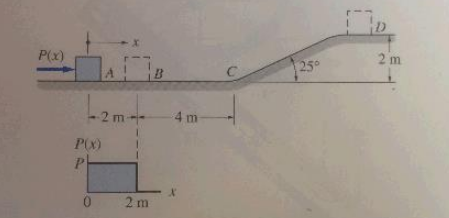# A 10 kg package, initially at rest, is propelled forward between A and B by a constant force P....

## Question:

A 10 kg package, initially at rest, is propelled forward between A and B by a constant force P. Neglecting friction, find the smallest value of P for which the package will reach D.## Conservation of energy:

Conservation of energy can be only balance; it can't be created neither destroyed. Energy changes from one state to another state. In energy balance equation of a system the initial kinetic energy and potential energy and work done is equal to the final kinetic energy and potential energy.

## Answer and Explanation:

Given data:

• The mass is: {eq}m = 10\;{\rm{kg}} {/eq}
• The vertical height from ground to point D is: {eq}h = 2\;\;{\rm{m}} {/eq}

The expression for the energy using work energy theorem is,

{eq}P\left( {6\;{\rm{m}}} \right) = \dfrac{1}{2}m{v^2} {/eq}

The expression for the conservation of energy at point C and D is,

{eq}\dfrac{1}{2}m{v^2} = mgh {/eq}

Here, {eq}g {/eq} is acceleration due to gravity.

Substitute the known values in above expression.

{eq}P\left( {6\;{\rm{m}}} \right) = mgh {/eq}

Substitute the given values in above expression.

{eq}\begin{align*} P\left( {6\;{\rm{m}}} \right) &= \left( {10\;{\rm{kg}}} \right)\left( {9.81\;{{\rm{m}} {\left/ {\vphantom {{\rm{m}} {{{\rm{s}}^2}}}} \right. } {{{\rm{s}}^2}}}} \right)2\;{\rm{m}}\\ P &= 32.7\;{{{\rm{kg}} \cdot {\rm{m}}} {\left/ {\vphantom {{{\rm{kg}} \cdot {\rm{m}}} {{{\rm{s}}^2}}}} \right. } {{{\rm{s}}^2}}}\left( {\dfrac{{1\;{\rm{N}}}}{{1\;{{{\rm{kg}} \cdot {\rm{m}}} {\left/ {\vphantom {{{\rm{kg}} \cdot {\rm{m}}} {{{\rm{s}}^2}}}} \right. } {{{\rm{s}}^2}}}}}} \right)\\ &= 32.7\;{\rm{N}} \end{align*} {/eq}

Thus, the smallest value of {eq}P {/eq} is {eq}32.7\;{\rm{N}} {/eq}.

#### Learn more about this topic:What is Energy Conservation? - Definition, Process & Examples

from ICSE Environmental Science: Study Guide & Syllabus

Chapter 1 / Lesson 6
92K